With these tools, you will be able to calculate very quickly one of the underlying functions with an easy data entry method.

The calculators in this section are practical tools that have informational value and do not replace any consulting nature and do not constitute an indicated or implied guarantee.

## Design toolsThis calculation tool measures the inside diameter of a pipe, flow rate or velocity. By entering two of the three required parameters, it will automatically calculate the third.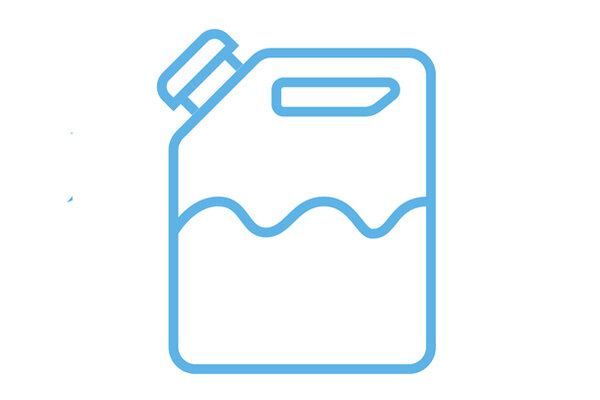The purpose of this calculation tool is to obtain the volume and time required to empty a tank, knowing its shape, dimensions and height of the liquid it contains.This calculation tool allows you to calculate the power absorbed by a pump knowing the characteristics of the pipe and the fluid, the pressures and the pressure drop. The values of some parameters such as the efficiency of the pump, the density of the liquid and the roughness of the pipe are already suggested as they are very common, but obviously they can be modified in case of different operating conditions.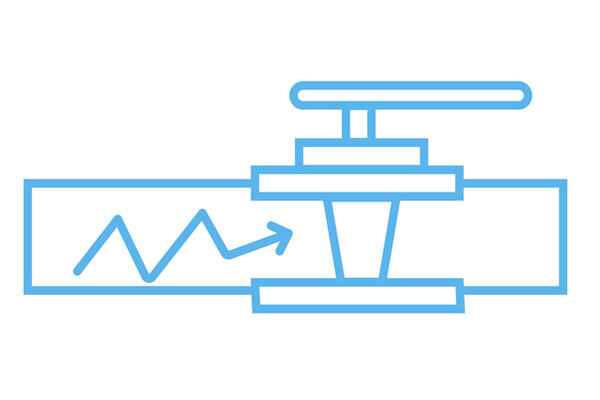Water hammer is a temporary increase in pressure when flow stops or starts rapidly. This tool calculates maximum pressure based on pipe characteristics, valve closing time, fluid velocity and inlet pressure.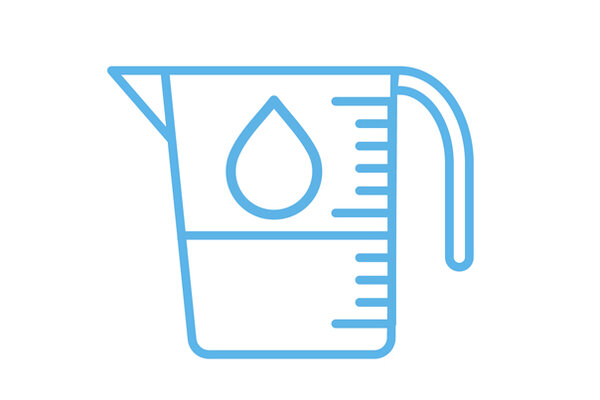The purpose of this calculation tool is to measure the flow rate inside a valve by knowing its flow coefficient, the difference between outlet and inlet pressure and the specific weight of the liquid compared to that of water at 15°C.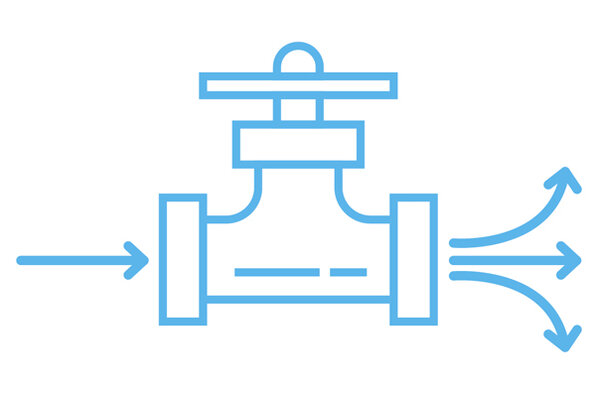This calculator measures the flow coefficient of a valve by knowing its flow rate, the difference between outlet and inlet pressure and the specific gravity of the liquid compared to that of water at 15°C.This calculation tool allows you to measure the pressure difference inside a valve by knowing the flow coefficient, the flow rate, and the specific weight of the liquid compared to that of water at 15°C.This tool graphically represents the relationship between nominal pressure and temperature based on the type of material used and the SDR. The graph obtained was calculated considering a useful life of 25 years.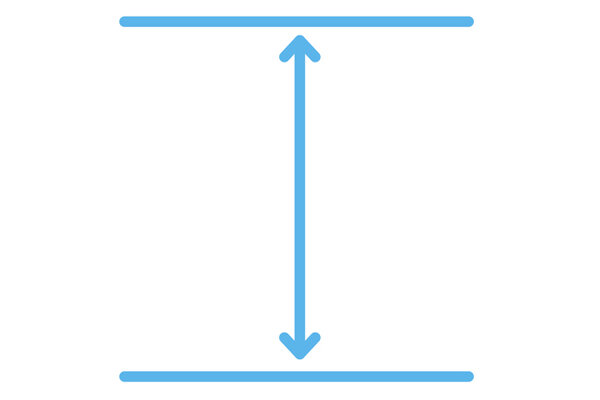Calculation of the flow rate based on the size of the valve Based on the operating conditions of the process, this tool allows you to calculate the flow rate of the fluid that can flow inside the valve used. By entering the type of valve chosen, the inlet and outlet pressure and the fluid properties as input, the tool shows the characteristic graph. In this way it is possible to identify the range of flow rates in which it is recommended to operate according to the degree of opening of the valve. To obtain optimal regulation, it is recommended to size the valve so as to keep the flow rate within the central area of the curves.This calculation tool allows you to calculate the total head losses within a system, i.e. distributed and localized head losses. Distributed pressure drops occur when the energy loss is generated by the surface friction developed between the liquid and the pipe walls. In localized leaks, on the other hand, the loss of energy is generated by shape friction, by a change of direction of the fluid in the presence of valves or fittings.

## Tools for installation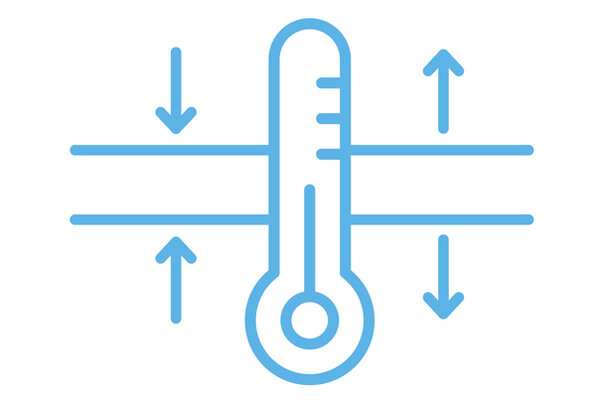Due to temperature changes in a system, pipes can expand and contract. To prevent breakage of the fasteners, compensators are usually used or expansion rings or direction changes are made. This tool allows us to calculate the correct parameters to prevent the potential expansion or contraction of our systems.This calculator provides the tightening torque and minimum bolt length to install flanges correctly.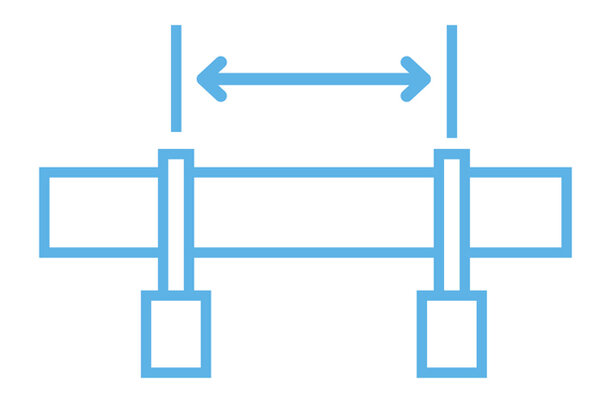With this calculation tool it is possible to define the minimum distance between the supports of a pipe according to the characteristics of the pipe itself, the temperature and the fluid flowing inside.This calculator indicates the main parameters for butt weldingThis calculator indicates the main parameters for socket welding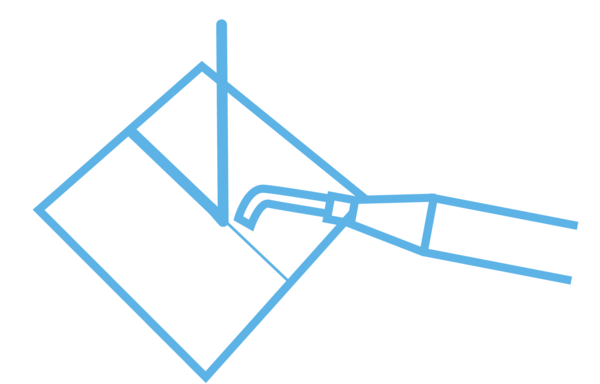This calculator indicates the main parameters for hot gas welding